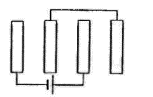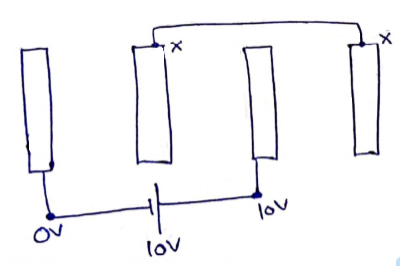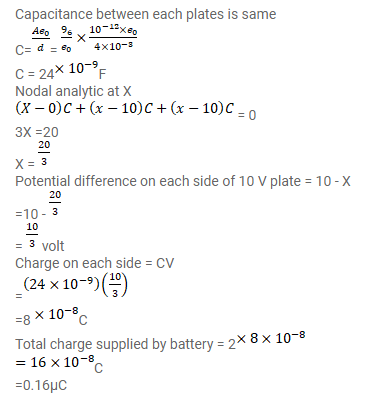# Each of the plates shown in figure has surface areaQuestion:

Each of the plates shown in figure has surface area

$\left(96 / \varepsilon_{0}\right) \times 10^{-1} F-m$

on one side and the separation between the consecutive plates is $4.0 \mathrm{~mm}$. The emf of the battery connected is 10 volts. Find the magnitude of the charge supplied by the battery to each of the plates connected to it.Solution: